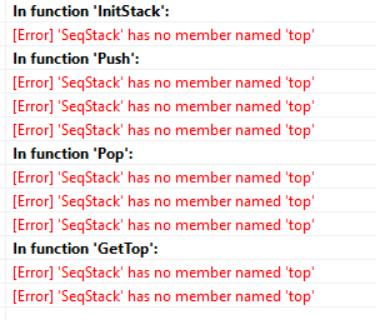【数据结构】用栈实现的带括号的多位数四则运算器

# 2.程序中出现如图所示错误，麻烦大佬指点一下原因!`````` #include <stdio.h>
#include <stdlib.h>

#define TRUE 1
#define FALSE 0
#define Stack_Size 50

typedef char StackElementType;
typedef struct
{
StackElementType elem[Stack_Size];
int Top;
}SeqStack;

void InitStack(SeqStack *S);
int Push(SeqStack *S,StackElementType x);
int Pop(SeqStack *S,StackElementType *x);
int GetTop(SeqStack *S,StackElementType *x);
StackElementType Compare(StackElementType ch1,StackElementType ch2);
StackElementType Execute(StackElementType a,StackElementType op,StackElementType b);

int main()
{
printf("%c",ExpEvaluation());
return(0);
}
StackElementType ExpEvaluation()
{
SeqStack OPTR,OVS;
StackElementType n;
StackElementType a,b,ch,op,x,v;
InitStack(&OPTR);
InitStack(&OVS);
Push(&OPTR,'#');
printf("Please input an expression(Ending with #):");
ch=getchar();//为方便，所以数字也以字符形式来存储
while(ch!='#'||GetTop(&OPTR,&x)!='#')
{
if(ch>='0'&&ch<='9')//不是操作符，是操作数，进OVS栈
{
int temp;
temp=ch-'0';//先把当前操作数从字符变为数字
ch=getchar();
while(ch>='0'&&ch<='9')//继续判断下一位是否为操作数
{
temp=temp*10+ch-'0';
ch=getchar();
}
Push(&OVS,temp);

}
else
switch(Compare(ch,GetTop(&OPTR,&x)))
{
case '>':Push(&OPTR,ch);
scanf("%d",&ch);
case '=':Pop(&OPTR,&op);ch=getchar();break;//脱括号
case '<':Pop(&OPTR,&op);//形成运算
Pop(&OVS,&b);
Pop(&OVS,&a);
v=Execute(a,op,b);
//此处需要将v转换为字符型并且入站
Push(&OVS,v);
break;
}
}
v=GetTop(&OVS,&x);
return(v);
}

//初始化
void InitStack(SeqStack *S)
{
/*构造一个空栈S*/
S->top=-1;
}
//进栈
int Push(SeqStack *S,StackElementType x)
{
if(S->top==Stack_Size-1)
return(FALSE);
S->top++;
S->elem[S->top]=x;
return(TRUE);
}
//出栈
int Pop(SeqStack *S,StackElementType *x)
{
if(S->top==-1)
return(FALSE);
*x=S->elem[S->top];
S->top--;
return(TRUE);
}
//读栈顶
int GetTop(SeqStack *S,StackElementType *x)
{
if(S->top==-1)
return(FALSE);
else
{
*x=S->elem[S->top];
return(TRUE);
}
}

//比较优先级，返回'>','<','='
StackElementType Compare(StackElementType ch1,StackElementType ch2)
//ch1为还未进栈的运算符，ch2为当前运算符栈顶元素
{
//'('未入栈时，优先级最高，入栈后，优先级最低
switch(ch1)
{
case '(':ch1=6;break;
case '*':
case '/':ch1=5;break;
case '+':
case '-':ch1=4;break;
case ')':ch1=3;break;
}
switch(ch2)
{
case '*':
case '/':ch2=5;break;
case '+':
case '-':ch2=4;break;
case ')':ch2=3;break;
case '(':ch2=2;break;
}
if(ch1>ch2)
return('>');
else if(ch1=ch2)
return('=');
else
return('<');
}
StackElementType Execute(StackElementType a,StackElementType op,StackElementType b)
{
double v;
switch(op)
{
case '+':v=(double)a+(double)b;break;
case '-':v=(double)a-(double)b;break;
case '*':v=(double)a*(double)b;break;
case '/':v=(double)a/(double)b;break;
}
return((StackElementType)v);
}

``````

1个回答

C语言是大小写敏感的语言
typedef struct
{
StackElementType elem[Stack_Size];
int Top;
}SeqStack;

S->top++;